GFG App
Open AppBrowser
Continue

Quadrilaterals can be defined as types of polygons that have four sides, four vertices, and four angles, along with a pair of diagonals. The Sum of interior angles of quadrilaterals is 360°. There are various kinds of quadrilaterals; the quadrilateral shapes themselves explain what type of quadrilateral it is. As the name itself suggest, the word is a combination of two Latin words ‘Quadri‘ means a variant of four, and ‘latus‘ means side.

A quadrilateral is defined as a polygon having 4 sides, 4 angles, and 4 vertices. The quadrilateral is a type of polygon in which the sides are defined in a proper pattern. For example, in the diagram below, the quadrilateral can be defined as ABCD, ADCB, BCDA, CDAB, etc. It cannot be defined as ACBD or BDAC. Here, the quadrilateral’s sides are AB, BC, CD, and DA, and the diagonals are AC and BD.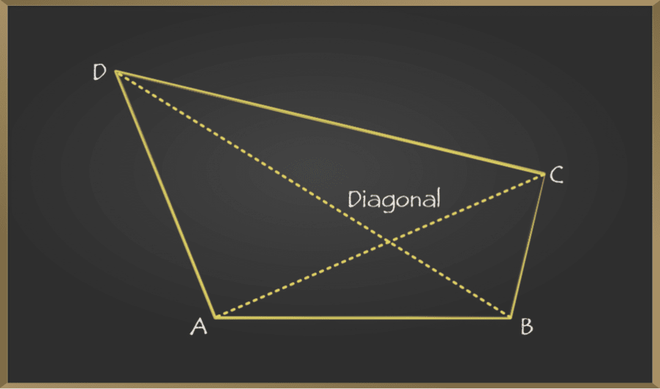Quadrilateral has several properties that make it different from a regular polygon. The different types of quadrilaterals have special properties to the quadrilateral. However, the properties of quadrilaterals are all valid for the different types of quadrilaterals. The properties of a quadrilateral are:

• A quadrilateral has 4 sides.
• A quadrilateral has 4 vertices.
• A quadrilateral has 4 angles.
• A quadrilateral has 2 diagonals.
• The sum of internal angles of a quadrilateral is 360°.

There are different types of quadrilateral, and all the types have different shapes. Quadrilateral shapes explain a lot about their properties; just by looking at the quadrilateral shapes, one can tell the difference and qualities among them.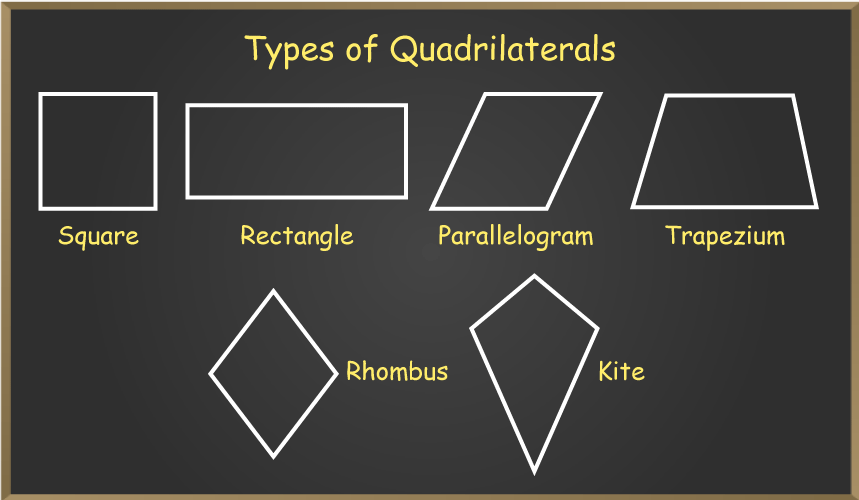Based on their properties, types of quadrilaterals are subdivided into other groups, and the main subdivision of the quadrilaterals is between convex and concave quadrilaterals. These concave and convex quadrilaterals can be further classified into their subdivision.

Quadrilaterals that have one interior angle greater than 180° and one diagonal lies outside the quadrilateral are called concave quadrilaterals. One of the examples of a concave quadrilateral is a Dart. It is a quadrilateral with bilateral symmetry like a kite, but with a reflex interior angle.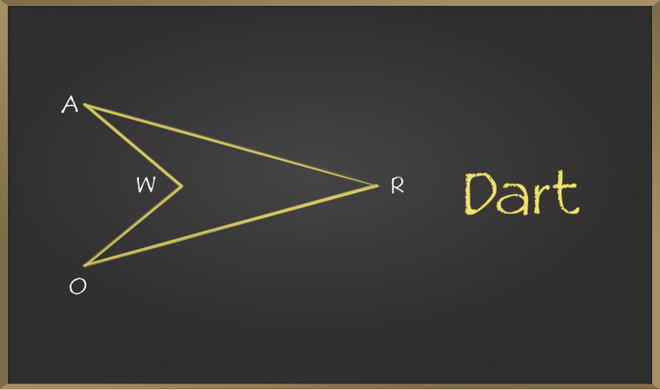Here, in the image given below, one of the interior angles of the quadrilateral is 210°, which is greater than 180°. Therefore,  the quadrilateral is a concave quadrilateral.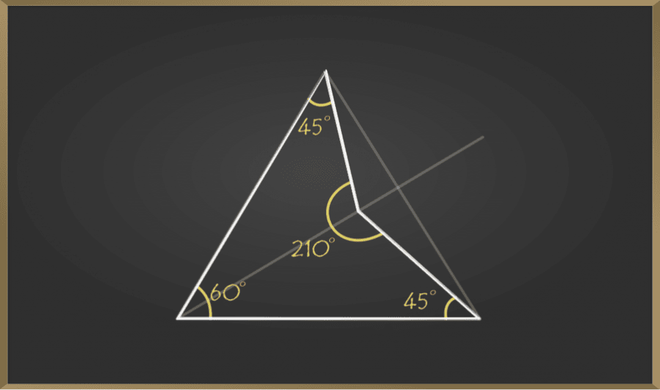Quadrilaterals that have all four interior angles less than 180° are called concave quadrilaterals. There are various types of Convex Quadrilaterals:

• Trapezium
• Kite
• Parallelogram
• Rectangle
• Rhombus
• Square

### Trapezium

A trapezium is a quadrilateral that has one pair of opposite sides parallel. In a regular trapezium, non-parallel sides are equal, and its base angles are equal. The area of trapezium is 1/2 × Sum of parallel sides × Distance between them.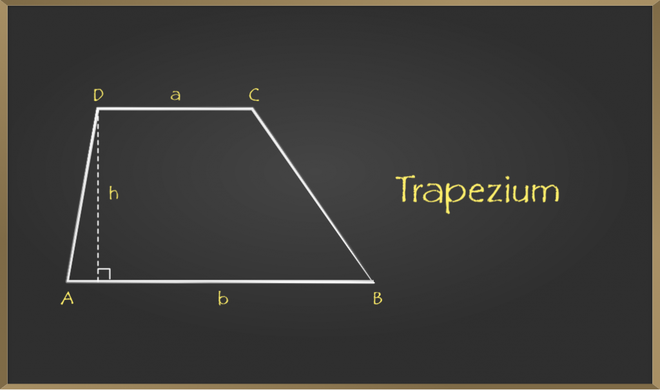• The sides of the trapezium that are parallel to each other are known as the bases of trapezium. In the above image, AB and CD are the base of the trapezium.
• The sides of the trapezium that are non-parallel are called the legs. In the above image, AD and BC are the legs.

### Kite

Kite has two pairs of equal adjacent sides and one pair of opposite angles equal. Diagonals of kites intersect perpendicularly. The longest diagonal of the kite bisects the smaller one.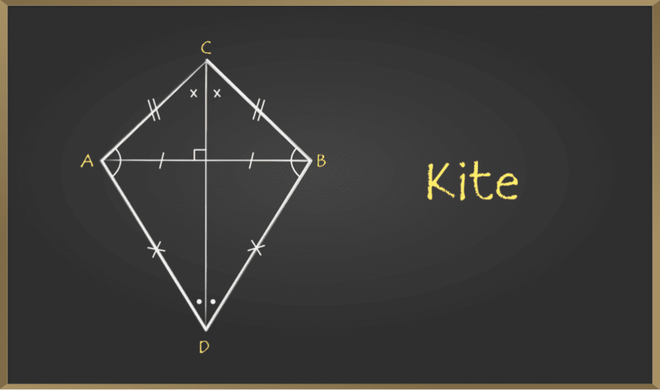• A kite has two pairs of equal adjacent sides. For example, AC = BC and AD = BD.
• The interior opposite angles that are obtuse are equal; here, ∠A = ∠B.
• The diagonals of a kite are perpendicular to each other; here, AB is perpendicular to CD.
• The longer diagonal of the kite bisects the shorter diagonal. Here, CD bisects AB.

### Parallelogram

A quadrilateral whose opposite sides are equal and parallel is called a Parallelogram. Opposite angles of a Parallelogram are equal, and its diagonals bisect each other.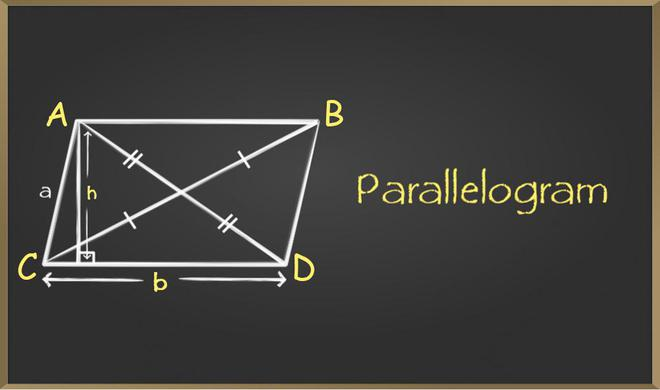• The opposite sides of a parallelogram are parallel and equal. In the above example, AB and CD are parallel and equal, and AC and BD are parallel and equal.
• The opposite angles in a parallelogram are equal. ∠A = ∠D and ∠B = ∠C.
• The diagonals of a parallelogram bisect each other.

### Rectangle

A quadrilateral whose opposite sides are equal and parallel and all the interior angles equal to 90° is defined as Rectangle. Diagonals of rectangles bisect each other. Note that all the rectangles are parallelograms, but the reverse of this is not true. The area of rectangle is length × breadth.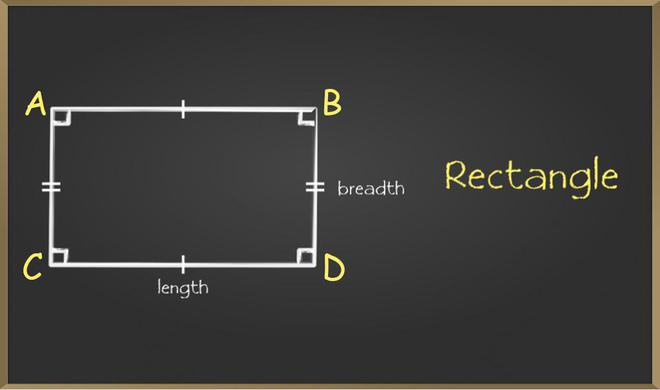• The opposite sides of a rectangle are parallel and equal. In the above example, AB and CD are parallel and equal, and AC and BD are parallel and equal.
• All 4 angles of a rectangle are equal and are equal to 90°. ∠A = ∠B = ∠C = ∠D = 90°.
• The diagonals of a rectangle bisect each other and the diagonals of a rectangle are equal, here, AD = BC.

### Rhombus

A quadrilateral that has all sides equal and opposite sides parallel is called Rhombus. Opposite angles of a rhombus are equal, and diagonals of the Rhombus bisect each other perpendicularly. Note all rhombus are parallelograms, but the reverse of this is not true.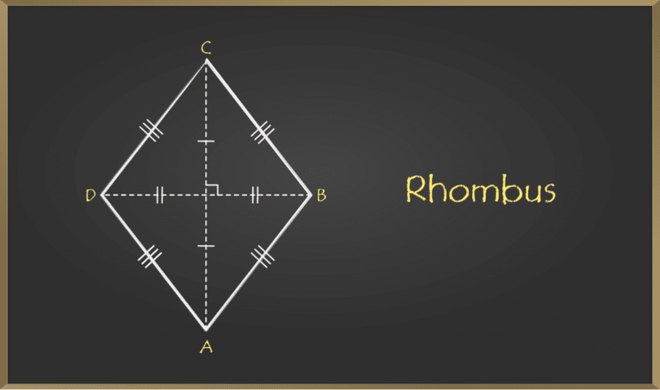• All 4 sides of a rhombus are equal. AB = BC = CD = AD.
• The opposite sides of a rhombus are parallel and equal. In the image above, AB is parallel to CD and AD is parallel to BC.
• The diagonals of a rhombus Bisect each other at 90°.

### Square

A quadrilateral that has all sides equal and opposite sides parallel and all interior angles equal to 90° is called a Square. Diagonals of squares bisect each other perpendicularly. Note that all squares are rhombus but not vice-versa.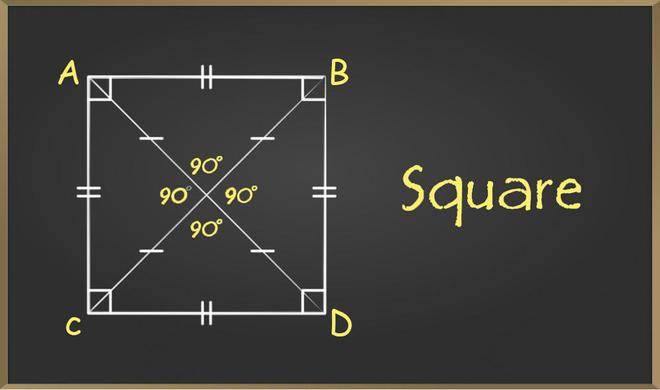• All four sides of a square are equal to each other.
• The interior angles of a square are 90°.
• The diagonal of a square bisect each other at 90°.
• The opposite sides are parallel, and the adjacent sides are perpendicular in a square.

The area of a quadrilateral is defined as the amount of space covered by a quadrilateral in a two-dimensional space. The different types of quadrilaterals have different formulae for their respective area. Below given table shows the formula for the area of each type of quadrilateral: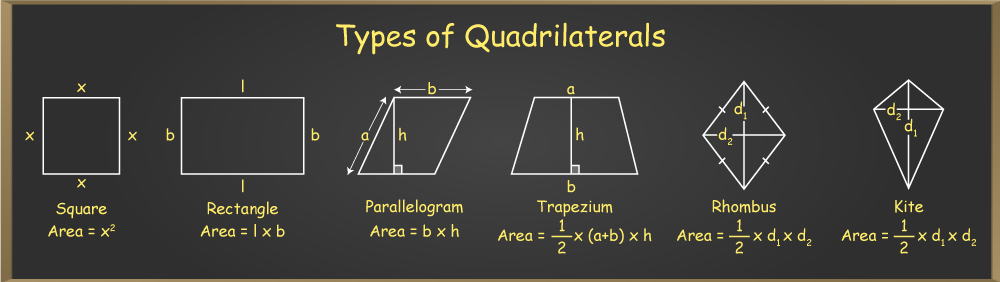The perimeter of a quadrilateral is the sum of all its sides. For different quadrilateral shapes, the perimeter formula is different as they portray different characteristics. Below are the formulas for different quadrilateral shapes.

Example 1: The perimeter of quadrilateral ABCD is 46 units. AB = x + 7, BC = 2x + 3, CD = 3x – 8, and DA = 4x – 6. Find the length of the shortest side of the quadrilateral.

Solution

Perimeter = Sum of all sides

= 46 = 10x – 4 or [x = 5]

That gives, AB = 12 units, BC = 13 units, CD = 7 units, DC = 14 units

Hence length of shortest side is 7 units (i.e. CD).

Example 2: Given a trapezoid ABCD (AB || DC) with median EF. AB = 3x – 5, CD = 2x -1 and EF = 2x + 1. Find the value of EF.

Solution

We know that the Median of the trapezoid is half the sum of its bases.

= EF = (AB + CD) / 2

= 4x + 2 = 5x – 6  or [x = 8]

Therefore EF = 2x + 1 = 2(8) + 1 => EF = 17 units.

Example 3: In a Parallelogram, adjacent angles are in the ratio of 1:2. Find the measures of all angles of this Parallelogram.

Solution:

Let the adjacent angle be x and 2x.

We know that in of a Parallelogram adjacent angles are supplementary.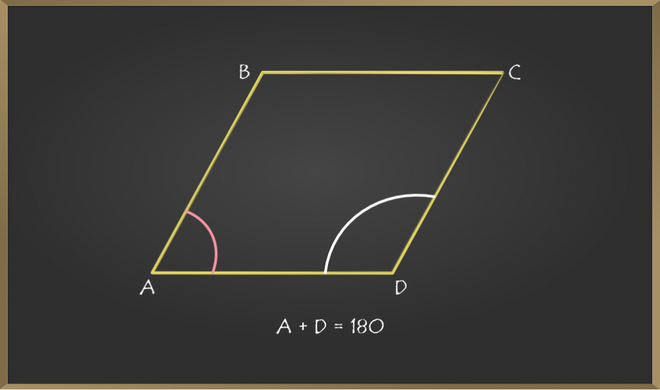= x + 2x = 180° or [x = 60°]

Also, opposite angles are equal in a Parallelogram.

Therefore measures of each angles are 60°, 120°, 60°, 120°.

Example 4: Perimeter of a rhombus is 52 units, and the length of one of its diagonal is 24 units. Find the length of another diagonal.

Solution:

Given: Perimeter = 52 unit.

Length of diagonal (say AC) = 24 Units.

We know that, rhombus has its all four sides equal.

= AB + BC + CD + DA = 52.

= 4(AB) = 52 = AB = 13 units.

Also, diagonals of Rhombus bisects each other perpendicularly. Hence in given picture AE = EC and BE = ED and ∠AEB = 90°.

Applying Pythagoras theorem in ∆AEB,(∠AEB = 90°)

= (AB)2 = (AE)2 + (EB)2

= (13)2 = (12)2 + (EB)2

= (EB)2 = 169 – 144 = 25

= EB  =  5 units.

Since, AC = 2 × EB = 10 units.

Therefore, required length of another diagonal of rhombus is 10 units.

Question 1: What is a quadrilateral?

A quadrilateral can be defined as a type of polygon that has four sides, four vertices, and four angles along with a pair of diagonals. The Sum of interior angles of a quadrilateral is 360°.

Question 2: Explain the Golden quadrilateral.

Golden quadrilateral is the famous term given to the national highway that connects the major states and the industrial, agriculateral, and cultural centres of India. On the map, the shape of the highway is the shape of a quadrilateral.

Question 3: What is the sum of the interior angles of a quadrilateral?

The sum of the interior angles of a quadrilateral is 360°. The same case applies to all quadrilateral shapes.

Question 4: How to find the area of a quadrilateral?

The area of a quadrilateral is defined as the space covered by the quadrilateral shape in 2-dimensional space. The different types of quadrilateral have different formula for area according to their properties. For example, the area of square is a2 where, a is the side length, the area of rectangle is l × b, where l and b are the length and breadth respectively, etc.

Question 5: What is a cyclic quadrilateral?